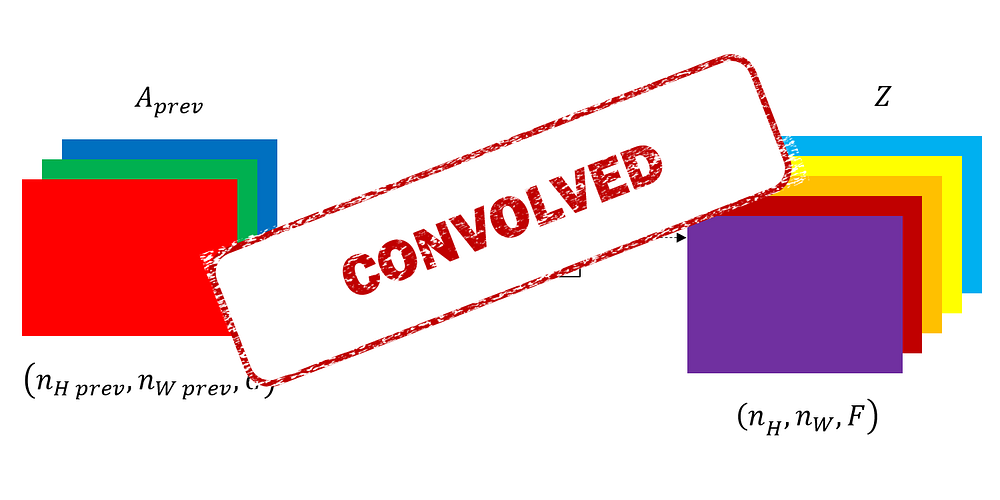# Forward and backward propagations for 2D Convolutional layers

Source: Deep Learning on Medium# Convolutional layer

The layer transforms the output of the previous layer A_prev of height n_H_prev, width n_W_prev and C channels into the variable Z of height n_H, width n_W and of F channels.

The parameters of this layer are:

• F kernels (or filters) defined by their weights w_{i,j,c}^f and biases b^f
• Kernel sizes (k1, k2) explained above
• An activation function
• Strides (s1, s2) which defines the step on which the kernel is applied on the input image
• Paddings p1, p2 which define the number of zero that we add on the borders of A_prev

# Forward propagation

The convolutional layer forwards the padded input; therefore, we consider A_prev_pad for the convolution.

The equations of forward propagation are then:

# Backward propagation

Backward propagation has three goals:

• Propagate the error from a layer to the previous one
• Compute the derivative of the error with respect to the weights
• Compute the derivative of the error with respect to the biases

## Notation

For ease of notation, we define: